# Rhombus + area of shape - examples - page 2

1. DiamondRhombus has side 17 cm and and one of diagonal 22 cm long. Calculate its area.
2. Rhombus A2p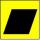Rhombus area is 13 cm2 and its height is 5cm long . Determine a perimeter of this rhombus.
3. Diamond diagonalsCalculate the diamond's diagonal lengths if its content is 156 cm2 and the side length is 13 cm.
4. Strip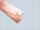From 5.9 cm wide strip should be cut rhombus with area 28 cm2. How long will be its side?
5. RhombusPQRS is a rhombus. Given that PQ=3 cm & height of rhombus is given 2 cm. Calculate its area.
6. Rhombus and diagonalsThe a rhombus area is 150 cm2 and the ratio of the diagonals is 3:4. Calculate the length of its height.
7. Rhombus 4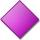Circumference of the rhombus is 44 cm, its height is 89 mm long. Calculate its content area.
8. Diagonals of diamondFind the area and circumference of the diamond ABCD with 15m and 11m diagonals.
9. Acreage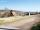Plot has a diamond shape, its side is 25.6 m long and the distance of the opposite sides is 22.2 meters. Calculate its acreage.
10. KiteJohn a kite, which is diamond shaped. Its diagonals are 60 cm long and 90 cm long. Calculate: a) the diamond side b) how much paper John needs to make a kite if he needs paper on both sides and needs 5% of the paper for bending.
11. Diagonals of a rhombusOne of the diagonals of a rhombus is twice as the other. If the sum of the lengths of the diagonals is 24, find the area of the rhombus.
12. Diamond perimeter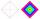Calculate the diamond circumference which area is 288 cm square and one diagonal has a size of 124 cm.
13. Rhombus sidesfind the sides of a rhombus, it area is 550 cm square and altitude is 55cm
14. DiagonalsGiven a rhombus ABCD with a diagonalsl length of 8 cm and 12 cm. Calculate the side length and content of the rhombus.
15. RhombusThe rhombus has diagonal lengths of 4.2cm and 3.4cm. Calculate the length of the sides of the rhombus and its height
16. Diamond diagonalsCalculate the diamonds' diagonals lengths if the diamond area is 156 cm square and the side length is 13 cm.
17. A squareA square with length of diagonals 12cm give: a) Calculate the area of a square b) rhombus with the same area as the square, has one diagonal with length of 16 cm. Calculate the length of the other diagonal.
18. Diamond diagonalsFind the diamond diagonal's lengths if the area is 156 cm2 and side is 13 cm long.
19. Diagonals of a rhombus 2One diagonal of a rhombus is greater than other by 4 cm . If the area of the rhombus is 96 cm2, find the side of the rhombus.
20. Diagonals of the rhombusHow long are the diagonals e, f in the diamond, if its side is 5 cm long and its area is 20 cm2?

Do you have an interesting mathematical example that you can't solve it? Enter it, and we can try to solve it.

To this e-mail address, we will reply solution; solved examples are also published here. Please enter e-mail correctly and check whether you don't have a full mailbox.